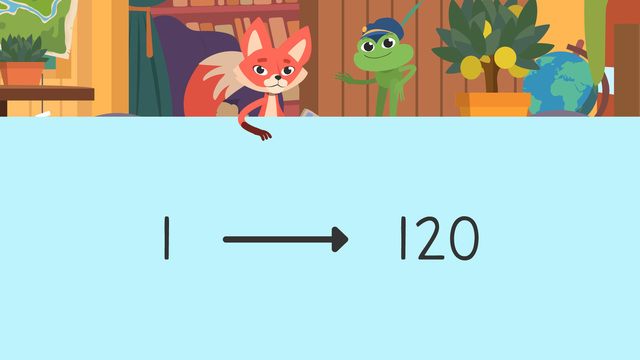# Counting to 120Rating

Be the first to give a rating!
The authorsTeam Digital
Counting to 120
CSS.MATH.CONTENT.1.NBT.A.1

## Counting to 120

In this text, we will learn how to count to 120 by 1s, starting at any number less than one hundred twenty.

The numbers one hundred to one hundred twenty follow a pattern when counting just like the numbers one to one hundred do! We can use a hundreds chart to help us see these patterns.## Counting to 120 – Hundreds Chart

Let’s have a closer look at a hundreds chart. One through ten always go in this order in the ones place. Then, one through ten follow the same pattern in all the rows that follow! Only the number in the tens place changes as we move down the rows.In this row, the tens place always has a one and the number in the ones place follows the one through ten pattern: eleven, twelve, thirteen, and so on. Instead of the last number changing to ten, it now changes from nineteen to twenty.## Counting to 120 – Numbers above Hundred

The same patterns follow when we count above one hundred! This time we just say 'one hundred' first! One hundred-one, one hundred-two, one hundred-three and so on.Remember to add the hundredths place in a place value chart, whenever you are counting numbers above 100. The number one hundred one looks like this when we put it into a place value chart:

Hundreds Tens Ones
1 0 1

## Counting to 120 – Finding Missing Numbers

To find a missing number in the chart, go to the number before it. Then, start counting-on using our pattern to figure it out!## Counting to 120 – Summary

If you want to count numbers from 1 to 120, you can use a hundreds chart to help you illustrate the patterns that numbers between 1 and 120 show. Below are the most important facts about numbers from 1 to 120:

• The numbers one through one hundred twenty follow a pattern when counting. The pattern starts with one through ten. Then, the tens place changes by one as we go down each row.

• The same patterns work when we count ABOVE one hundred. This time we just say 'one hundred' before we start!

• To find a missing number in a one hundred twenty chart, go to the number before it.

• Then, start counting on using our pattern to find what's missing!

Have you practiced yet? On this website, you can also find interactive exercises, worksheets and more activities to help you practice counting to 120. You’ll say “I can count to 120” in no time!

## Counting to 120 exercise

Would you like to apply the knowledge you’ve learned? You can review and practice it with the tasks for the video Counting to 120.
• ### Use the 120 chart to figure out the missing number.

Hints

Remember, to find the missing number, start at the number before and count on.

Remember, look for patterns in the rows and columns.

Solution

We start at the number before the missing number, 101. 101, 102. By counting up, we discover the missing number is 102. This follows the patterns of the other rows and columns.

• ### Identify the missing numbers.

Hints

Remember, read the number before, and use the pattern to count and figure out the missing number.

Look at this chart. Find the number before and count on to determine the missing numbers.

Solution

By counting on, we identify the missing numbers. 111, 112, 113, 114, 115, 116, 117, 118. Even though we are above 100, the pattern stays the same.

• ### Complete the row.

Hints

Remember, in this row, the tens place always has a two, and the number in the ones place changes.

Look at this example of a different row to see the pattern.

Solution

To fill in this row, we follow the pattern in the ones place. We look at the number before, and count on. 21, 22, 23, 24, 25, 26, 27, 28, 29. The pattern continues in the ones place.

• ### Complete the column.

Hints

Remember, only the number in the tens place changes as we move down the rows.

Look at the number before, and the number after. Count on to find what number is missing.

Solution

The missing numbers are 32, 42, 62, 72, and 82! As we moved down the rows of the hundreds chart, only the tens place changed.

• ### How many chocolate flies?

Hints

Remember, the same pattern continues past 100. Start at the number before, and count on.

Remember, we use the chart to solve for the missing number. In this chart, 34 is the number before this missing number. We count on to solve for the missing number. 34, 35. The missing number is 35.

Solution

Henry won 109 chocolate flies! The number before was 108, and we counted on. 108, 109.

• ### Complete the column past 100.

Hints

Remember, look at the number before the missing number, and the number after. Count on to find the missing number.

Remember, the ones place changes in rows, and the tens place changes in columns.

Solution

The same patterns continue when we count above 100. We found the missing numbers by going to the number before it, and counting on. For example, the first missing number was 65. We went to 64, and counted on to get 65. The same pattern was used to find the missing number 119. We start at the number before, 118, and count on to get 119. Now Skylar and Henry can complete the puzzle!